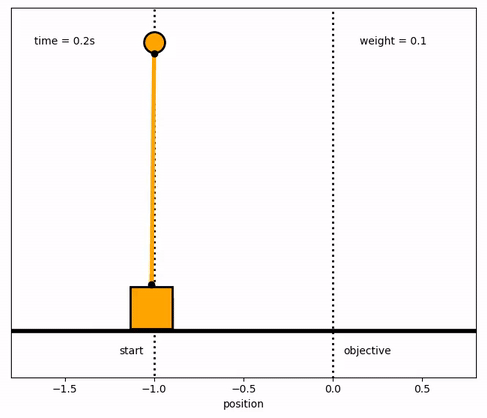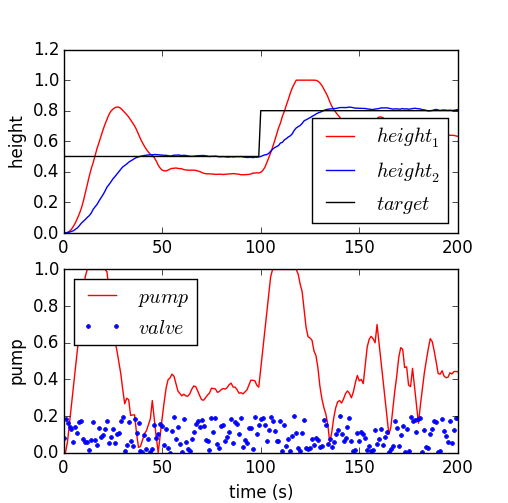## Main.FinalExam History

March 21, 2019, at 03:59 PM by 10.35.117.63 -

(:toggle hide gekko button show="Show GEKKO (Python) Code":) (:div id=gekko:) (:source lang=python:) import numpy as np from gekko import GEKKO

1. Check solution with GEKKO

m = GEKKO() m.time=[0,1] u = m.Var(value=1) x = m.Var(value=0) m.Obj((x-3)**2) m.Equation(5*x.dt()==-x+2*u) m.options.IMODE = 6 m.options.NODES = 3 m.solve()

1. Matrix solution for Orthogonal Collocation on Finite Elements
2. min (x-3)^2
3. s.t. 5 * dx/dt = -x + 2 * u
4. Equations 1-2 (subject to equations at each node)
5. 5 * xdot1 = -x1 + 2*u1
6. 5 * xdot2 = -x2 + 2*u2
7. Equations 3-4 (collocation equations)
8. t2 * 0.75 * xdot1 - t2 * 0.25 * xdot2 = x1 - x0
9. t2 * 1.00 * xdot1 = x2 - x0
10. Equations 5-6 (derivative of objective = 0)
11. 2 * (x1-3) = 0
12. 2 * (x2-3) = 0
13. Rearrange to put all variables on left-hand side
14. Equations 1-2 (subject to equations at each node)
15. 5 * xdot1 + x1 - 2*u1 = 0
16. 5 * xdot2 + x2 - 2*u2 = 0
17. Equations 3-4 (collocation equations)
18. t2 * 0.75 * xdot1 - t2 * 0.25 * xdot2 - x1 = -x0
19. t2 * 1.00 * xdot1 - x2 = -x0
20. Equations 5-6 (derivative of objective = 0)
21. 2 * x1 = 6
22. 2 * x2 = 6
23. Set-up and solve A y = b
24. y = [xdot1 xdot2 x1 x2 u1 u2]
25. Matrix A

A = np.array()

1. Column vector b

b = np.array([0,0,0,0,6,6])

1. Solve A y = b as y = A^-1 * b

ymat = np.linalg.solve(A,b)

print('Variables with Orthogonal Collocation') print(['u1 = ' + str(ymat)]) print(['u2 = ' + str(ymat) + ' (Matrix) vs ' + str(u.value[-1]) + ' (GEKKO)']) print(['x1 = ' + str(ymat)]) print(['x2 = ' + str(ymat) + ' (Matrix) vs ' + str(x.value[-1]) + ' (GEKKO)']) print(' ') print('Derivatives with Orthogonal Collocation') print(['d(x11)/dt = ' + str(ymat)]) print(['d(x21)/dt = ' + str(ymat)]) (:sourceend:) (:divend:)

March 23, 2018, at 03:42 PM by 10.5.113.167 -
Changed lines 30-41 from:

#### Solution 3Dual Tank: Step Test for Model Identification (Simulink/Excel)Dual Tank: Step Test for Model Identification (Python/Excel)

(:html:) <iframe width="560" height="315" src="https://www.youtube.com/embed/U7uyj9BaNKg" frameborder="0" allowfullscreen></iframe> (:htmlend:)Dual Tank: Linear MPC Solution (MATLAB/Simulink)Dual Tank: Linear MPC Solution (Python)
to:See Level Control
Deleted lines 33-34:

(:html:) (:htmlend:)

March 23, 2018, at 01:46 PM by 10.5.113.167 -
Changed line 1 from:

(:title 2015 Final Exam for Dynamic Optimization:)

to:

(:title Practice Final Exam:)

March 22, 2018, at 08:45 PM by 10.5.113.167 -
Changed line 26 from:
to:March 22, 2018, at 08:44 PM by 10.5.113.167 -
Changed line 26 from:to:
March 22, 2018, at 12:31 AM by 10.37.35.33 -March 22, 2018, at 12:04 AM by 10.37.35.33 -

March 22, 2018, at 12:03 AM by 10.37.35.33 -
Changed lines 10-11 from:

to:

#### Solution 1

Changed lines 20-21 from:
to:

#### Solution 2See Inverted Pendulum Solution

#### Solution 3

March 22, 2018, at 12:02 AM by 10.37.35.33 -
Changed lines 20-24 from:Inverted Pendulum: Solution Files

(:html:) <iframe width="560" height="315" src="https://www.youtube.com/embed/iW52CoG33pk" frameborder="0" allowfullscreen></iframe> (:htmlend:)

to:
March 26, 2016, at 01:02 AM by 10.5.113.125 -March 23, 2016, at 03:06 PM by 45.56.3.173 -
Changed line 29 from:Dual Tank: Step Test for Model Identification (Python)
to:Dual Tank: Step Test for Model Identification (Python/Excel)
March 23, 2016, at 01:22 PM by 45.56.3.173 -
Changed line 28 from:Dual Tank: Step Test for Model Identification (MATLAB/Simulink/Excel)
to:Dual Tank: Step Test for Model Identification (Simulink/Excel)
March 23, 2016, at 01:21 PM by 45.56.3.173 -
Changed lines 28-29 from:Dual Tank: Step Test for Model Identification Δ
to:Dual Tank: Step Test for Model Identification (MATLAB/Simulink/Excel)Dual Tank: Step Test for Model Identification (Python)
Changed lines 35-36 from:Dual Tank: Linear MPC Solution
to:Dual Tank: Linear MPC Solution (MATLAB/Simulink)Dual Tank: Linear MPC Solution (Python)
March 23, 2016, at 01:18 PM by 45.56.3.173 -Dual Tank: Step Test for Model Identification Δ

(:html:) <iframe width="560" height="315" src="https://www.youtube.com/embed/U7uyj9BaNKg" frameborder="0" allowfullscreen></iframe> (:htmlend:)

March 21, 2016, at 03:45 PM by 45.56.3.173 -
Changed line 28 from:Dual Tank MPC: Solution Files Δ
to:Dual Tank: Linear MPC Solution
February 09, 2016, at 09:55 PM by 174.148.97.76 -
Changed line 1 from:

(:title Final Exam for Dynamic Optimization:)

to:

(:title 2015 Final Exam for Dynamic Optimization:)

Changed line 3 from:

(:description Final exam for the graduate level course on dynamic estimation and optimization.:)

to:

(:description Final exam for the graduate level course on dynamic estimation and optimization in 2015.:)

February 09, 2016, at 09:53 PM by 174.148.97.76 -
Changed line 5 from:

to:

#### Final Exam 2015

June 15, 2015, at 02:27 PM by 45.56.3.184 -
Changed lines 12-13 from:Final Exam Solution Key (PDF) Δ
to:KKT Conditions for Dynamic Optimization: Solution Files

(:html:) <iframe width="560" height="315" src="https://www.youtube.com/embed/n4OzENKziR4" frameborder="0" allowfullscreen></iframe> (:htmlend:)

Changed lines 20-21 from:KKT Conditions for Dynamic Optimization Solution Files
to:Inverted Pendulum: Solution Files
Changed line 23 from:

<iframe width="560" height="315" src="https://www.youtube.com/embed/n4OzENKziR4" frameborder="0" allowfullscreen></iframe>

to:

<iframe width="560" height="315" src="https://www.youtube.com/embed/iW52CoG33pk" frameborder="0" allowfullscreen></iframe>

Changed lines 28-36 from:Inverted Pendulum Solution Files

(:html:) <iframe width="560" height="315" src="https://www.youtube.com/embed/iW52CoG33pk" frameborder="0" allowfullscreen></iframe> (:htmlend:)Dual Tank MPC Solution Files Δ
to:Dual Tank MPC: Solution Files Δ
June 15, 2015, at 02:17 PM by 45.56.3.184 -

#### SolutionFinal Exam Solution Key (PDF) ΔKKT Conditions for Dynamic Optimization Solution Files

(:html:) <iframe width="560" height="315" src="https://www.youtube.com/embed/n4OzENKziR4" frameborder="0" allowfullscreen></iframe> (:htmlend:)Inverted Pendulum Solution Files

(:html:) <iframe width="560" height="315" src="https://www.youtube.com/embed/iW52CoG33pk" frameborder="0" allowfullscreen></iframe> (:htmlend:)Dual Tank MPC Solution Files Δ

(:html:) (:htmlend:)

June 05, 2015, at 11:15 PM by 10.5.113.170 -
Changed lines 1-3 from:

(:title Midterm Exam for Dynamic Optimization:) (:keywords Python, MATLAB, Simulink, nonlinear control, model predictive control, exam, midterm:) (:description Mid-term exam for dynamic estimation and optimization as a graduate-level course.:)

to:

(:title Final Exam for Dynamic Optimization:) (:keywords Python, MATLAB, Simulink, nonlinear control, model predictive control, exam, final:) (:description Final exam for the graduate level course on dynamic estimation and optimization.:)

June 05, 2015, at 08:29 PM by 10.10.144.142 -
Changed lines 7-8 from:Final Exam ΔFinal Exam Files Δ
to:Final ExamFinal Exam Files
June 05, 2015, at 04:57 PM by 45.56.3.184 -
Changed lines 7-8 from:Final ExamFinal Exam Files
to:Final Exam ΔFinal Exam Files Δ
June 05, 2015, at 04:56 PM by 45.56.3.184 -
May 18, 2015, at 04:06 PM by 45.56.3.184 -Final ExamFinal Exam Files